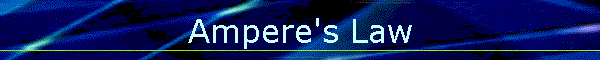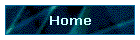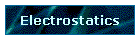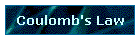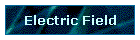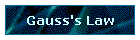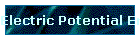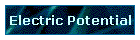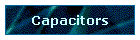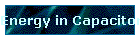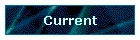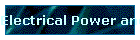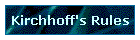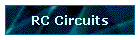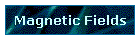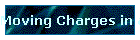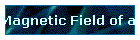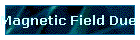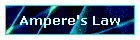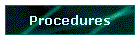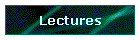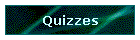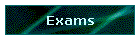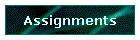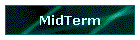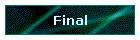Phys 4, Section 2                                                    Feb 25, 2002   Ampere's Law For a closed loop of perimeter L with a current I enclosed in the loop, Ampere's law asserts: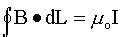Displacement Current Ampere's Law as given above fails when applied to a circuit with a charging capacitor.  Recall that C =  Q/V and for a flat-plate capacitor, C = eoA/d.  Using q, v and i for instantaneous values of charge, potential and current, respectively, q = Cv = (eoA/d)(Ed) = eoEA = eoFE, with FE representing the electric flux.  Since i = dq/dt, then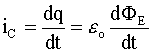is an expression for the current flowing into the capacitor. This expression was extended by James Maxwell to cover the displacement current flowing through the empty space between the capacitor plates. Note that this displacement current does not involve the motion of charge, but rather the rate of change of an E-field. Note also that the displacement current between the plates is equal to the real current of moving charge flowing into the capacitor. Maxwell extended Ampere's law to include displacement currents: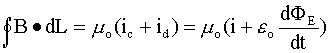Note that since Φ = ∫ E•dA , a displacement current density jD = iD/A can be defined as: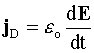Last modified on February 27, 2002